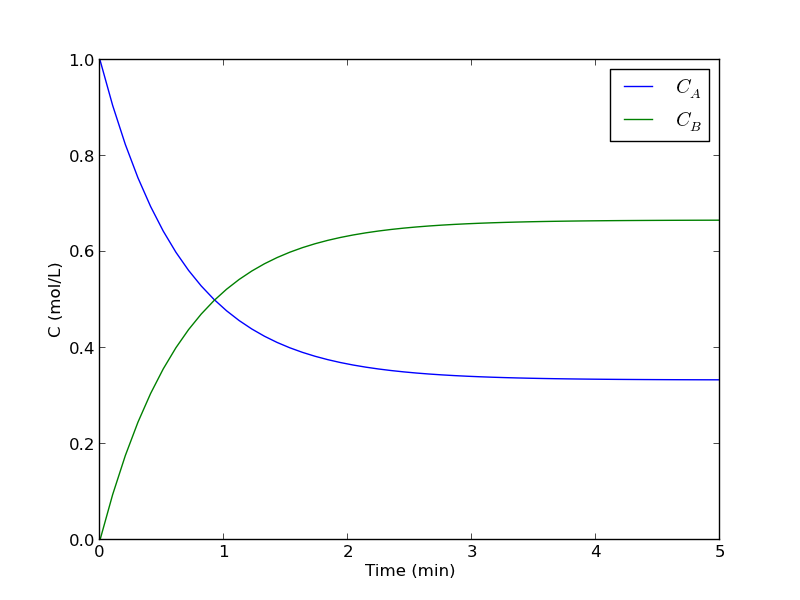Time dependent concentration in a first order reversible reaction in a batch reactor

| categories: ode | tags: reaction engineering

Given this reaction $$A \rightleftharpoons B$$, with these rate laws:

forward rate law: $$-r_a = k_1 C_A$$

backward rate law: $$-r_b = k_{-1} C_B$$

plot the concentration of A vs. time. This example illustrates a set of coupled first order ODES.

from scipy.integrate import odeint
import numpy as np

def myode(C, t):
# ra = -k1*Ca
# rb = -k_1*Cb
# net rate for production of A:  ra - rb
# net rate for production of B: -ra + rb

k1 = 1   # 1/min;
k_1 = 0.5   # 1/min;

Ca = C
Cb = C

ra = -k1 * Ca
rb = -k_1 * Cb

dCbdt = -ra + rb

return dCdt

tspan = np.linspace(0, 5)

init = [1, 0]  # mol/L
C = odeint(myode, init, tspan)

Ca = C[:,0]
Cb = C[:,1]

import matplotlib.pyplot as plt
plt.plot(tspan, Ca, tspan, Cb)
plt.xlabel('Time (min)')
plt.ylabel('C (mol/L)')
plt.legend(['$C_A$', '$C_B$'])
plt.savefig('images/reversible-batch.png')That is it. The main difference between this and Matlab is the order of arguments in odeint is different, and the ode function has differently ordered arguments.

org-mode source

Solving integral equations with fsolve

| categories: nonlinear algebra | tags: reaction engineering

Occasionally we have integral equations we need to solve in engineering problems, for example, the volume of plug flow reactor can be defined by this equation: $$V = \int_{Fa(V=0)}^{Fa} \frac{1}{r_a} dFa$$ where $$r_a$$ is the rate law. Suppose we know the reactor volume is 100 L, the inlet molar flow of A is 1 mol/L, the volumetric flow is 10 L/min, and $$r_a = -k Ca$$, with $$k=0.23$$ 1/min. What is the exit molar flow rate? We need to solve the following equation:

$$100 = \int_{Fa(V=0)}^{Fa} \frac{1}{-k Fa/\nu} dFa$$

We start by creating a function handle that describes the integrand. We can use this function in the quad command to evaluate the integral.

import numpy as np
from scipy.optimize import fsolve

k = 0.23
nu = 10.0
Fao = 1.0

def integrand(Fa):
return -1.0 / (k * Fa / nu)

def func(Fa):
return 100.0 - integral

vfunc = np.vectorize(func)

We will need an initial guess, so we make a plot of our function to get an idea.

import matplotlib.pyplot as plt

f = np.linspace(0.01, 1)
plt.plot(f, vfunc(f))
plt.xlabel('Molar flow rate')
plt.savefig('images/integral-eqn-guess.png')
plt.show()
>>> >>> [<matplotlib.lines.Line2D object at 0x964a910>]
<matplotlib.text.Text object at 0x961fe50>Now we can see a zero is near Fa = 0.1, so we proceed to solve the equation.

Fa_guess = 0.1
Fa_exit, = fsolve(vfunc, Fa_guess)
print 'The exit concentration is {0:1.2f} mol/L'.format(Fa_exit / nu)
>>> The exit concentration is 0.01 mol/L

1 Summary notes

This example seemed a little easier in Matlab, where the quad function seemed to get automatically vectorized. Here we had to do it by hand.

org-mode source

Integrating a batch reactor design equation

| categories: integration | tags: reaction engineering

For a constant volume batch reactor where $$A \rightarrow B$$ at a rate of $$-r_A = k C_A^2$$, we derive the following design equation for the length of time required to achieve a particular level of conversion :

$$t(X) = \frac{1}{k C_{A0}} \int_{X=0}^X \frac{dX}{(1-X)^2}$$

if $$k = 10^{-3}$$ L/mol/s and $$C_{A0}$$ = 1 mol/L, estimate the time to achieve 90% conversion.

We could analytically solve the integral and evaluate it, but instead we will numerically evaluate it using scipy.integrate.quad. This function returns two values: the evaluated integral, and an estimate of the absolute error in the answer.

def integrand(X):
k = 1.0e-3
Ca0 = 1.0  # mol/L
return 1./(k*Ca0)*(1./(1-X)**2)

sol, abserr = quad(integrand, 0, 0.9)
print 't = {0} seconds ({1} hours)'.format(sol, sol/3600)
print 'Estimated absolute error = {0}'.format(abserr)
t = 9000.0 seconds (2.5 hours)
Estimated absolute error = 2.12203274482e-07

You can see the estimate error is very small compared to the solution.

org-mode source

Integrating the batch reactor mole balance

| categories: ode | tags: reaction engineering

An alternative approach of evaluating an integral is to integrate a differential equation. For the batch reactor, the differential equation that describes conversion as a function of time is:

$$\frac{dX}{dt} = -r_A V/N_{A0}$$.

Given a value of initial concentration, or volume and initial number of moles of A, we can integrate this ODE to find the conversion at some later time. We assume that $$X(t=0)=0$$. We will integrate the ODE over a time span of 0 to 10,000 seconds.

from scipy.integrate import odeint
import numpy as np
import matplotlib.pyplot as plt

k = 1.0e-3
Ca0 = 1.0  # mol/L

def func(X, t):
ra = -k * (Ca0 * (1 - X))**2
return -ra / Ca0

X0 = 0
tspan = np.linspace(0,10000)

sol = odeint(func, X0, tspan)
plt.plot(tspan,sol)
plt.xlabel('Time (sec)')
plt.ylabel('Conversion')
plt.savefig('images/2013-01-06-batch-conversion.png')You can read off of this figure to find the time required to achieve a particular conversion.